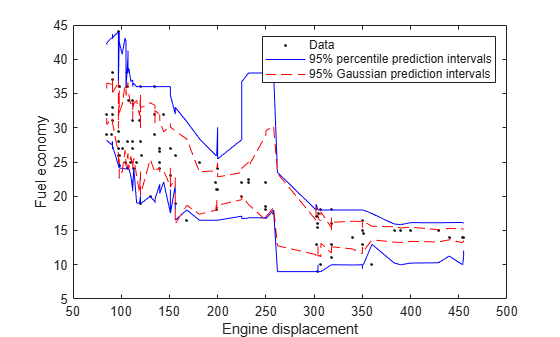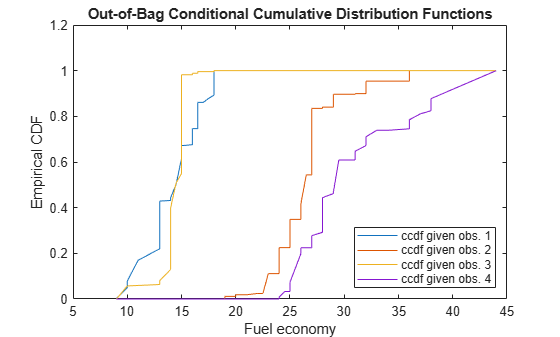# oobQuantilePredict

Class: TreeBagger

Quantile predictions for out-of-bag observations from bag of regression trees

## Syntax

``YFit = oobQuantilePredict(Mdl)``
``YFit = oobQuantilePredict(Mdl,Name,Value)``
``````[YFit,YW] = oobQuantilePredict(___)``````

## Description

example

````YFit = oobQuantilePredict(Mdl)` returns a vector of medians of the predicted responses at all out-of-bag observations in `Mdl.X`, the predictor data, and using `Mdl`, which is a bag of regression trees. `Mdl` must be a `TreeBagger` model object and `Mdl.OOBIndices` must be nonempty.```

example

````YFit = oobQuantilePredict(Mdl,Name,Value)` uses additional options specified by one or more `Name,Value` pair arguments. For example, specify quantile probabilities or trees to include for quantile estimation.```

example

``````[YFit,YW] = oobQuantilePredict(___)``` also returns a sparse matrix of response weights using any of the previous syntaxes.```

## Input Arguments

expand all

Bag of regression trees, specified as a `TreeBagger` model object created by `TreeBagger`.

• The value of `Mdl.Method` must be `regression`.

• When you train `Mdl` using `TreeBagger`, you must specify the name-value pair `'OOBPrediction','on'`. Consequently, `TreeBagger` saves required out-of-bag observation index matrix in `Mdl.OOBIndices`.

### Name-Value Arguments

Specify optional pairs of arguments as `Name1=Value1,...,NameN=ValueN`, where `Name` is the argument name and `Value` is the corresponding value. Name-value arguments must appear after other arguments, but the order of the pairs does not matter.

Before R2021a, use commas to separate each name and value, and enclose `Name` in quotes.

Quantile probability, specified as the comma-separated pair consisting of `'Quantile'` and a numeric vector containing values in the interval [0,1]. For each observation (row) in `Mdl.X`, `oobQuantilePredict` estimates corresponding quantiles for all probabilities in `Quantile`.

Example: `'Quantile',[0 0.25 0.5 0.75 1]`

Data Types: `single` | `double`

Indices of trees to use in response estimation, specified as the comma-separated pair consisting of `'Trees'` and `'all'` or a numeric vector of positive integers. Indices correspond to the cells of `Mdl.Trees`; each cell therein contains a tree in the ensemble. The maximum value of `Trees` must be less than or equal to the number of trees in the ensemble (`Mdl.NumTrees`).

For `'all'`, `oobQuantilePredict` uses the indices `1:Mdl.NumTrees`.

Example: `'Trees',[1 10 Mdl.NumTrees]`

Data Types: `char` | `string` | `single` | `double`

Weights to attribute to responses from individual trees, specified as the comma-separated pair consisting of `'TreeWeights'` and a numeric vector of `numel(trees)` nonnegative values. `trees` is the value of the `Trees` name-value pair argument.

The default is `ones(size(trees))`.

Data Types: `single` | `double`

## Output Arguments

expand all

Estimated quantiles for out-of-bag observations, returned as an `n`-by-`numel(tau)` numeric matrix. `n` is the number of observations in the training data (`numel(Mdl.Y)`) and `tau` is the value of the `Quantile` name-value pair argument. That is, `YFit(j,k)` is the estimated `100*tau(k)` percentile of the response distribution given `X(j,:)` and using `Mdl`.

Response weights, returned as an n-by-n sparse matrix. n is the number of responses in the training data (`numel(Mdl.Y)`). `YW(:,j)` specifies the response weights for the observation in `Mdl.X(j,:)`.

`oobQuantilePredict` predicts quantiles using linear interpolation of the empirical cumulative distribution function (cdf). For a particular observation, you can use its response weights to estimate quantiles using alternative methods, such as approximating the cdf using kernel smoothing.

## Examples

expand all

Load the `carsmall` data set. Consider a model that predicts the fuel economy (in MPG) of a car given its engine displacement.

`load carsmall`

Train an ensemble of bagged regression trees using the entire data set. Specify 100 weak learners and save out-of-bag indices.

```rng(1); % For reproducibility Mdl = TreeBagger(100,Displacement,MPG,'Method','regression',... 'OOBPrediction','on');```

`Mdl` is a `TreeBagger` ensemble.

Perform quantile regression to predict the out-of-bag median fuel economy for all training observations.

`oobMedianMPG = oobQuantilePredict(Mdl);`

`oobMedianMPG` is an `n`-by-1 numeric vector of medians corresponding to the conditional distribution of the response given the sorted observations in `Mdl.X`. `n` is the number of observations, `size(Mdl.X,1)`.

Sort the observations in ascending order. Plot the observations and the estimated medians on the same figure. Compare the out-of-bag median and mean responses.

```[sX,idx] = sort(Mdl.X); oobMeanMPG = oobPredict(Mdl); figure; plot(Displacement,MPG,'k.'); hold on plot(sX,oobMedianMPG(idx)); plot(sX,oobMeanMPG(idx),'r--'); ylabel('Fuel economy'); xlabel('Engine displacement'); legend('Data','Out-of-bag median','Out-of-bag mean'); hold off;```Load the `carsmall` data set. Consider a model that predicts the fuel economy of a car (in MPG) given its engine displacement.

`load carsmall`

Train an ensemble of bagged regression trees using the entire data set. Specify 100 weak learners and save out-of-bag indices.

```rng(1); % For reproducibility Mdl = TreeBagger(100,Displacement,MPG,'Method','regression',... 'OOBPrediction','on');```

Perform quantile regression to predict the out-of-bag 2.5% and 97.5% percentiles.

`oobQuantPredInts = oobQuantilePredict(Mdl,'Quantile',[0.025,0.975]);`

`oobQuantPredInts` is an `n`-by-2 numeric matrix of prediction intervals corresponding to the out-of-bag observations in `Mdl.X`. `n` is number of observations, `size(Mdl.X,1)`. The first column contains the 2.5% percentiles and the second column contains the 97.5% percentiles.

Plot the observations and the estimated medians on the same figure. Compare the percentile prediction intervals and the 95% prediction intervals, assuming the conditional distribution of `MPG` is Gaussian.

```[oobMeanMPG,oobSTEMeanMPG] = oobPredict(Mdl); STDNPredInts = oobMeanMPG + [-1 1]*norminv(0.975).*oobSTEMeanMPG; [sX,idx] = sort(Mdl.X); figure; h1 = plot(Displacement,MPG,'k.'); hold on h2 = plot(sX,oobQuantPredInts(idx,:),'b'); h3 = plot(sX,STDNPredInts(idx,:),'r--'); ylabel('Fuel economy'); xlabel('Engine displacement'); legend([h1,h2(1),h3(1)],{'Data','95% percentile prediction intervals',... '95% Gaussian prediction intervals'}); hold off;```Load the `carsmall` data set. Consider a model that predicts the fuel economy of a car (in MPG) given its engine displacement.

`load carsmall`

Train an ensemble of bagged regression trees using the entire data set. Specify 100 weak learners and save the out-of-bag indices.

```rng(1); % For reproducibility Mdl = TreeBagger(100,Displacement,MPG,'Method','regression',... 'OOBPrediction','on');```

Estimate the out-of-bag response weights.

`[~,YW] = oobQuantilePredict(Mdl);`

`YW` is an n-by-n sparse matrix containing the response weights. `n` is the number of training observations, `numel(Y)`. The response weights for the observation in `Mdl.X(j,:)` are in `YW(:,j)`. Response weights are independent of any specified quantile probabilities.

Estimate the out-of-bag, conditional cumulative distribution function (ccdf) of the responses by:

1. Sorting the responses is ascending order, and then sorting the response weights using the indices induced by sorting the responses.

2. Computing the cumulative sums over each column of the sorted response weights.

```[sortY,sortIdx] = sort(Mdl.Y); cpdf = full(YW(sortIdx,:)); ccdf = cumsum(cpdf);```

`ccdf(:,j)` is the empirical out-of-bag ccdf of the response, given observation `j`.

Choose a random sample of four training observations. Plot the training sample and identify the chosen observations.

```[randX,idx] = datasample(Mdl.X,4); figure; plot(Mdl.X,Mdl.Y,'o'); hold on plot(randX,Mdl.Y(idx),'*','MarkerSize',10); text(randX-10,Mdl.Y(idx)+1.5,{'obs. 1' 'obs. 2' 'obs. 3' 'obs. 4'}); legend('Training Data','Chosen Observations'); xlabel('Engine displacement') ylabel('Fuel economy') hold off```Plot the out-of-bag ccdf for the four chosen responses in the same figure.

```figure; plot(sortY,ccdf(:,idx)); legend('ccdf given obs. 1','ccdf given obs. 2',... 'ccdf given obs. 3','ccdf given obs. 4',... 'Location','SouthEast') title('Out-of-Bag Conditional Cumulative Distribution Functions') xlabel('Fuel economy') ylabel('Empirical CDF')```expand all

## Algorithms

`oobQuantilePredict` estimates out-of-bag quantiles by applying `quantilePredict` to all observations in the training data (`Mdl.X`). For each observation, the method uses only the trees for which the observation is out-of-bag.

For observations that are in-bag for all trees in the ensemble, `oobQuantilePredict` assigns the sample quantile of the response data. In other words, `oobQuantilePredict` does not use quantile regression for out-of-bag observations. Instead, it assigns `quantile(Mdl.Y,tau)`, where `tau` is the value of the `Quantile` name-value pair argument.

## References

 Meinshausen, N. “Quantile Regression Forests.” Journal of Machine Learning Research, Vol. 7, 2006, pp. 983–999.

 Breiman, L. “Random Forests.” Machine Learning. Vol. 45, 2001, pp. 5–32.

## Version History

Introduced in R2016b